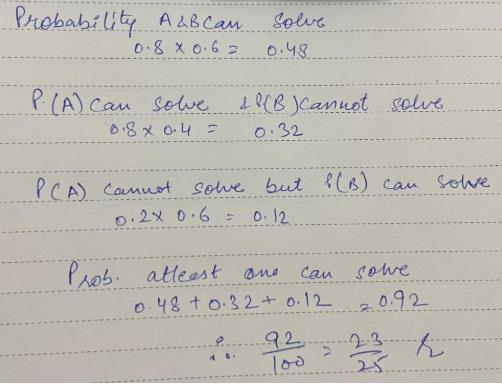# Test: Independent Events

## 10 Questions MCQ Test Mathematics (Maths) Class 12 | Test: Independent Events

Description
This mock test of Test: Independent Events for JEE helps you for every JEE entrance exam. This contains 10 Multiple Choice Questions for JEE Test: Independent Events (mcq) to study with solutions a complete question bank. The solved questions answers in this Test: Independent Events quiz give you a good mix of easy questions and tough questions. JEE students definitely take this Test: Independent Events exercise for a better result in the exam. You can find other Test: Independent Events extra questions, long questions & short questions for JEE on EduRev as well by searching above.
QUESTION: 1

### A die is tossed twice. The probability of getting 1, 2, 3 or 4 on the first toss and 4, 5, or 6 on the second toss is:​

Solution:

In each case, the sample space is given by S={1,2,3,4,5,6}.
Let E = event of getting a 1, 2, 3 or 4 on the first toss.
And, F = event of getting a 5, 6,or 7 on the second toss.
Then, P(E) = 4/6 = 2/3
and P(F) = 3/6 = 1/2
Clearly, E and F are independent events.
∴ required probability = P(E∩F) = P(E)×P(F) [∵ E and F are independent]
= 2/3 * 1/2 = 1/3

QUESTION: 2

Solution:
QUESTION: 3

### What is the probability of picking a spade from a normal pack of cards and rolling an odd number on a die?​

Solution:

Probability of getting odd no. while tossing a die = 3/6 = 1/2
Probability of getting spade from deck of 52 cards = 13/52 = 1/4
Probability of picking a spade from normal pack and rolling odd number = 1/2*1/4 = 1/8

QUESTION: 4

If A and B are two independent events, then

Solution:

P(A|B) = P(A∩B)/P(B)
=[P(A)P(B)]/P(B) [A is subset of B]
= P(A)

QUESTION: 5

Two parts A and B of a machine is manufactured by a firm. Out of 100 A’s 12 are likely to be defective and Out of 100 B’s 8 are likely to be defective. The probability that a machine manufactured by the firm is free from any defect is:​

Solution:

probability of getting good machine part from A = 88/100
probability of getting good machine part from B = 92/100
P(correction) = (88/100)(92/100)
= 506/625

*Multiple options can be correct
QUESTION: 6

A and B are two independent events. The probability that both A and B occur is 1/6 and the probability that neither of them occurs is 1/3. The probability of occurrence of A is.​

Solution:

The probability that both occur simultaneously is 1/6 and the probability that neither occurs is  1/3
Let P(A)=x, P(B)=y
Then P(A)×P(B) = ⅙ becomes xy = 1/6
​And [1−P(A)][1−P(B)]=1/3 becomes (1−x)(1−y)=1/3
​On Solving for x and y,
we get x=1/3 or x=1/2 which is the probability of occurrence of A.

QUESTION: 7

A and B are two independent events. The probability that both A and B occur is 1/6 and the probability that neither of them occurs is 1/3. The probability of occurrence of A is.​

Solution:

The probability that both occur simultaneously is 1/6 and the probability that neither occurs is  1/3
Let P(A)=x, P(B)=y
Then P(A)×P(B) = ⅙ becomes xy = 1/6
​And [1−P(A)][1−P(B)]=1/3 becomes (1−x)(1−y)=1/3
​On Solving for x and y,
we get x=1/3 or x=1/2 which is the probability of occurrence of A.

QUESTION: 8

Ashmit can solve 80% of the problem given in a book and Amisha can solve 70%. What is the probability that at least one of them will solve a problem selected at random from the book?

Solution:QUESTION: 9

If A and B are independent events, such that P(A ∪ B)= 0.7, P(B) = 0.5, then P(A) = ……​

Solution:

P(A ∪ B) = P(A) + P(B) - P(A)P(B)
= 0.7 = P(A) + 0.5 - 0.5 P(A)
0.2 = 0.5 P(A)
P(A) = ⅖
P(A) = 0.4

QUESTION: 10

A student can solve 70% problems of a book and second student solve 50% problem of same book. Find the probability that at least one of them will solve a selected problem from this book.​

Solution:

Probability that first and second student can solve
=0.7×0.5=0.35
Probability that first can solve and second cannot solve
=0.7×0.5=0.35
Probability that first cannot solve and Amisha can solve
=0.3×0.5=0.15
Therefore, probability that at least one of them will solve
=0.35+0.35+0.15 = 0.85
=> 85/100
= 17/20